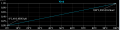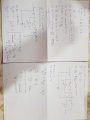# Temperature Sensor Design Required if possible.

#### wayneh

Joined Sep 9, 2010
17,201
Why not just use an LM35?

•spinnaker

Joined Mar 10, 2018
4,057
Homework problem ?

If so show some thoughts you have where to start.

Regards, Dana.

#### Elhakor5

Joined Dec 13, 2018
13
Why not just use an LM35?
Becasue thats a Course project and i tried alot to design it but im having some problems when simulating it, it gives wrong results.
so im trying to get more ideas#### crutschow

Joined Mar 14, 2008
31,584
Show the simulation results and the .asc file if it's from LTspice.
What are the component values in your schematic?

Last edited:

#### ericgibbs

Joined Jan 29, 2010
17,180
hi,
Where did you get that circuit.
E

#### crutschow

Joined Mar 14, 2008
31,584
That circuit should work.
The diode-connected transistor in the feedback loop has about a -2mV/°C change in voltage drop. (Diode-connected transistors tend to have a more ideal diode behavior as compared to a standard junction diode).
That op amp configuration generates a constant current through the transistor, as determined by the value of R1, so that the voltage change due to temperature does not affect its current, which would cause an error in the voltage.
So you select the values of R2 and R4 for an output op amp gain that gives the desired 10mV/°C change in Vo for the -2mV/°C change at the output of the left op amp.

You then select the value of R3 to cancel the offset voltage of the transistor of about 0.7v (typically to give 0V out at 0°C for an easy to interpret readout of 0 to 1V for 0 to 100°C).

These resistor values will need to be tweaked for the actual temperature coefficient and offset of the transistor you use.
[For the LT spice simulation I did, the 2N3906 coefficient (I used that PNP as recommended in a Linear Technology design paper) was ≈-1.86mV/°C and the offset at 0°C was ≈686mV at a bias current of 500μA as determined by R1].

Below are the results of my simulation.
I'll leave the resistor value calculations up to you.Last edited:
•wayneh

#### ericgibbs

Joined Jan 29, 2010
17,180
hi 5,
If you posted a link from where you sourced the circuit, it most likely has descriptive text, component types and calculation maths, that we could guide you through.

E

#### Elhakor5

Joined Dec 13, 2018
13
That circuit should work.
The diode-connected transistor in the feedback loop has about a -2mV/°C change in voltage drop. (Diode-connected transistors tend to have a more ideal diode behavior as compared to a standard junction diode).
That op amp configuration generates a constant current through the transistor, as determined by the value of R1, so that the voltage change due to temperature does not affect its current, which would cause an error in the voltage.
So you select the values of R2 and R4 for an output op amp gain that gives the desired 10mV/°C change in Vo for the -2mV/°C change at the output of the left op amp.

You then select the value of R3 to cancel the offset voltage of the transistor of about 0.7v (typically to give 0V out at 0°C for an easy to interpret readout of 0 to 1V for 0 to 100°C).

These resistor values will need to be tweaked for the actual temperature coefficient and offset of the transistor you use.
[For the LT spice simulation I did, the 2N3906 coefficient (I used that PNP as recommended in a Linear Technology design paper) was ≈-1.86mV/°C and the offset at 0°C was ≈686mV at a bias current of 500μA as determined by R1].

Below are the results of my simulation.
I'll leave the resistor value calculations up to you.View attachment 165718

#### Elhakor5

Joined Dec 13, 2018
13
hi 5,
If you posted a link from where you sourced the circuit, it most likely has descriptive text, component types and calculation maths, that we could guide you through.

E
This circuit is a course project which the professor gave it to us , I'm not sure where he got it from#### ericgibbs

Joined Jan 29, 2010
17,180
Hi,
The circuit will work OK as the sim in post #7 shows.
It would have been helpful if there had been some supporting text, so that we could give a specific answer.

Using a 2N3906 transistor, have a go at putting component values on that circuit and we can check your results.
As its a Homework assignment we do not give the answer, just guidance.
E

#### crutschow

Joined Mar 14, 2008
31,584
Did you understand what I said about the circuit?
If not, what questions do you have about the calculations?
Do you understand how op amps work?
We need to know your level of understanding.

Last edited:

#### Elhakor5

Joined Dec 13, 2018
13
Did you understand what I said about the circuit?
If not, what questions do you have about the calculations?
Do you understand how op amps work?
We need to know your level of understanding.
I know how op amps work, its just that when i try to analyze the circuit i cant make those temperature relations and im not sure what assumption on resistors i should make. and again thanks a lot for your time.#### ericgibbs

Joined Jan 29, 2010
17,180
hi 5,
R1 sets the nominal current in the trans/diode, keep the current low say 100uA for starters.
Look at the 2N2906 d/s and determine the Vbe value for the trans/diode at 100uA, it should approx 0.65V
So you now know the voltage input to the 2nd OPA via R2.
R3 is also is an input the 2nd OPA input.
As you want the output of the 2nd OPA to be close to zero volts at the lowest temperature, chose a value for R3,bearing in mind that one end is connected to -5v.

Assume a temperature range of say 0C thru +100C, so the 2nd OPA output should be from 0V thru +1V

E

#### ebp

Joined Feb 8, 2018
2,332
There are two distinctly different functions in that circuit, one for each op amp.

Can you partition the circuit into those functions?

#### crutschow

Joined Mar 14, 2008
31,584
I know how op amps work
Great.
If you do, then you should be able to tell me what the first op amp is doing.
What is the circuit configuration of that op amp (open or closed loop, inverting, non-inverting)?
its just that when i try to analyze the circuit i cant make those temperature relations
What "temperature relations"?
That tells me little about where you are having a problem.
Is it with how a transistor voltage changes with temperature, or how the op amp is used to generate the output voltage?
Or something else?

We are not going to spoon-feed you a solution.
You need to be more specific about where you are having a problem and show us what you have attempted.

Last edited:

#### Elhakor5

Joined Dec 13, 2018
13
Great.
If you do, then you should be able to tell me what the first op amp is doing.
What is the circuit configuration of that op amp (open or closed loop, inverting, non-inverting)?
What "temperature relations"?
That tells me little about where you are having a problem.
Is it with how a transistor voltage changes with temperature, or how the op amp is used to generate the output voltage?
Or something else?

We are not going to spoon-feed you a solution.
You need to be more specific about where you are having a problem and show us what you have attempted.
Hi again, Thx alot for your time , and yea actually i dont know what the first op amp with that transistor feedback do, all i tried to do until now is trying to analyze the circuit but i always get a negative value for R4.

#### ericgibbs

Joined Jan 29, 2010
17,180
hi 5,
Post what you calc the voltage output is from the 1st OPA,.?
E

If you have a diagram with some component values, please post it.

#### crutschow

Joined Mar 14, 2008
31,584
i dont know what the first op amp with that transistor feedback do
Okay.
So, ignoring R3 and the second op amp, if you apply a negative voltage to R1, what does the op amp do if the transistor in the negative feedback loop were just a resistor?
What is the current through that resistor?
Then substitute the transistor for the resistor and what is the result?
(Remember that an op amp with negative feedback, will do whatever it can at its output to keep the voltage between the two inputs at essentially 0V.)

#### Elhakor5

Joined Dec 13, 2018
13
hi 5,
Post what you calc the voltage output is from the 1st OPA,.?
E

If you have a diagram with some component values, please post it.
Okay.
So, ignoring R3 and the second op amp, if you apply a negative voltage to R1, what does the op amp do if the transistor in the negative feedback loop were just a resistor?
What is the current through that resistor?
Then substitute the transistor for the resistor and what is the result?
(Remember that an op amp with negative feedback, will do whatever it can at its output to keep the voltage between the two inputs at essentially 0V.)
ok This time i will post my solution and orcad simulation.